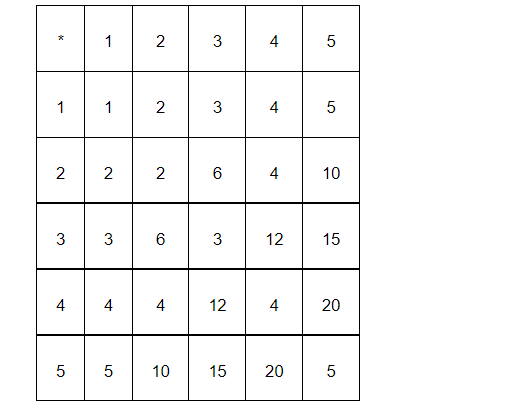# Is * defined on the set {1, 2, 3, 4, 5} by a * b = L.C.M.

Question:

Is * defined on the set {1, 2, 3, 4, 5} by * = L.C.M. of and a binary operation? Justify your answer.

Solution:

The operation * on the set A = {1, 2, 3, 4, 5} is defined as

a * b = L.C.M. of a and b.

Then, the operation table for the given operation * can be given as:It can be observed from the obtained table that:

$3 * 2=2 * 3=6 \notin A, 5^{*} 2=2 * 5=10 \notin A, 3^{*} 4=4^{*} 3=12 \notin A$

$3 * 5=5^{*} 3=15 \notin A, 4 * 5=5 * 4=20 \notin A$

Hence, the given operation * is not a binary operation.